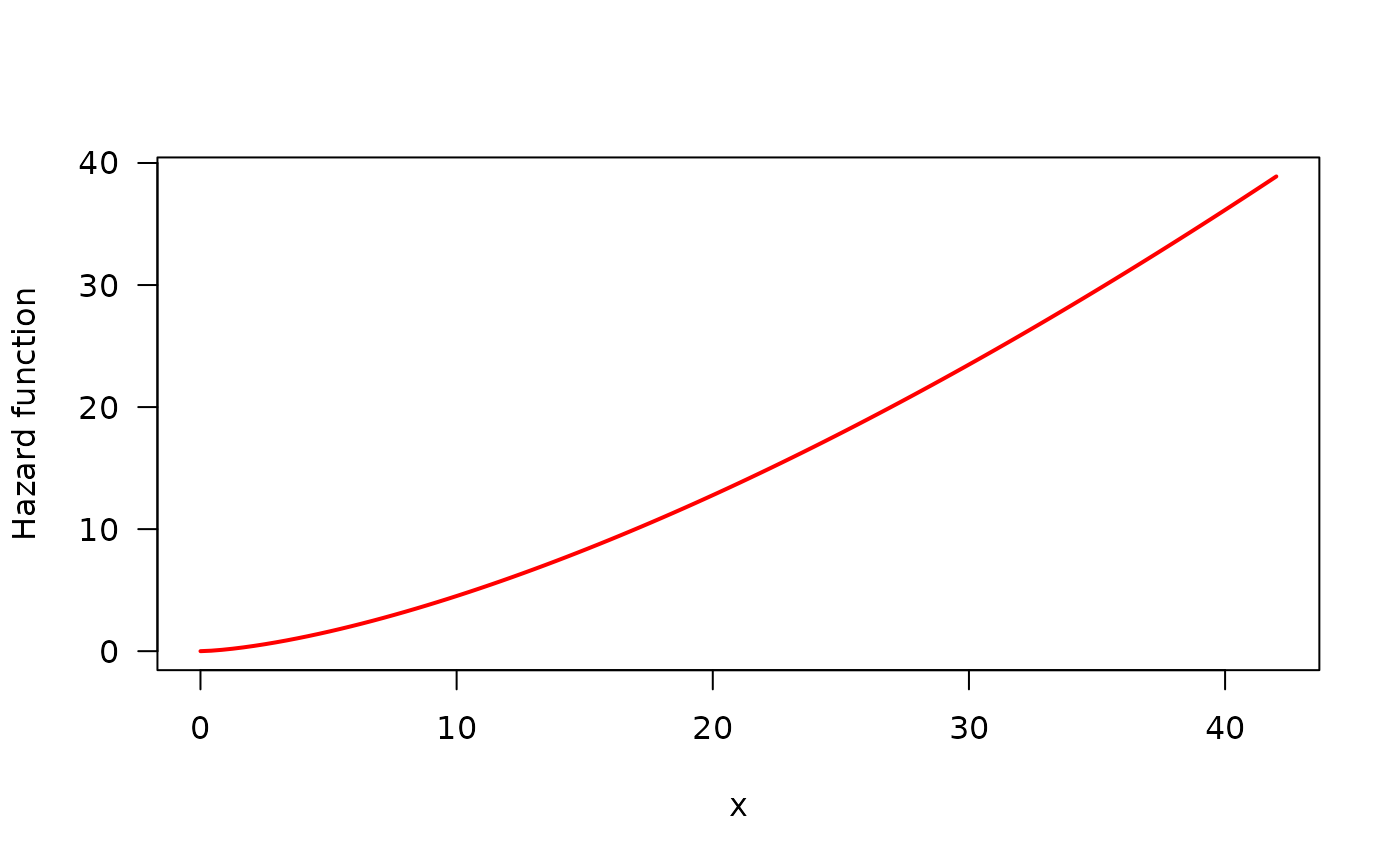This function can be used so as to estimate hazard shape corresponding to a given data set. This is a wrapper for TTTE_Analytical.

## Usage

TTT_hazard_shape(object, ...)

# S3 method for formula
TTT_hazard_shape(
formula,
data = NULL,
local_reg = loess.options(),
interpolation = interp.options(),
silent = FALSE,
...
)

# S3 method for EmpiricalTTT
TTT_hazard_shape(
object,
local_reg = loess.options(),
interpolation = interp.options(),
silent = FALSE,
...
)

## Arguments

object

An alternative way for getting the hazard shape estimation in passing directly the EmpiricalTTT object generated with TTTE_Analytical.

...

further arguments passed to TTTE_Analytical.

formula

An object of class formula with the response on the left of an operator ~. The right side must be 1.

data

an optional data frame containing the response variables. If data is not specified, the variables are taken from the environment from which TTT_hazard_shape is called.

local_reg

a list of control parameters for LOESS. See loess.options.

interpolation

a list of control parameters for interpolation function. See interp.options.

silent

logical. If TRUE, warnings of TTT_hazard_shape are suppressed.

## Details

This function performs a non-parametric estimation of the empirical total time on test (TTT) plot. Then, this estimated curve can be used so as to get suggestions about initial values and the search region for parameters based on hazard shape associated to the shape of empirical TTT plot.

Use Hazard_Shape function to get the results for shape estimation.

print.HazardShape, plot.HazardShape, TTTE_Analytical

## Author

Jaime Mosquera Gutiérrez jmosquerag@unal.edu.co

## Examples

#--------------------------------------------------------------------------------
# Example 1: Increasing hazard and its corresponding TTT statistic with
#            simulated data

hweibull <- function(x, shape, scale){
dweibull(x, shape, scale)/pweibull(x, shape, scale, lower.tail = FALSE)
}
curve(hweibull(x, shape = 2.5, scale = pi), from = 0, to = 42,
col = "red", ylab = "Hazard function", las = 1, lwd = 2)

y <- rweibull(n = 50, shape = 2.5, scale = pi)
status <- c(rep(1, 48), rep(0, 2))
my_initial_guess1 <- TTT_hazard_shape(Surv(y, status) ~ 1)
my_initial_guess1$hazard_type #>  "Increasing" #-------------------------------------------------------------------------------- # Example 2: Same example using an 'EmpiricalTTT' object y <- rweibull(n = 50, shape = 2.5, scale = pi) TTT_wei <- TTTE_Analytical(y ~ 1) my_initial_guess2 <- TTT_hazard_shape(TTT_wei) my_initial_guess2$hazard_type
#>  "Increasing"

#--------------------------------------------------------------------------------
# Example 3: Increasing hazard with simulated censored data

hweibull <- function(x, shape, scale){
dweibull(x, shape, scale)/pweibull(x, shape, scale, lower.tail = FALSE)
}
curve(hweibull(x, shape = 2.5, scale = pi), from = 0, to = 42,
col = "red", ylab = "Hazard function", las = 1, lwd = 2)y <- rweibull(n = 50, shape = 2.5, scale = pi)
y <- sort(y)
status <- c(rep(1, 45), rep(0, 5))
my_initial_guess1 <- TTT_hazard_shape(Surv(y, status) ~ 1)
my_initial_guess1\$hazard_type
#>  "Increasing"

#--------------------------------------------------------------------------------